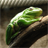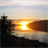# Move polyline or polygon geometry to a new centroid

2433
8
02-22-2017 02:59 AMOccasional Contributor II

I want to move polyline geometry to a new point programmatically.

``<!DOCTYPE html><html>  <head>    <meta http-equiv="Content-Type" content="text/html; charset=utf-8">    <meta name="viewport" content="width=device-width,user-scalable=no">    <meta name="viewport" content="initial-scale=1, maximum-scale=1,user-scalable=no">    <title>Maps Electrical Power Distribtution</title>        <link rel="stylesheet" href="https://js.arcgis.com/3.19/dijit/themes/nihilo/nihilo.css">    <link rel="stylesheet" href="https://js.arcgis.com/3.19/esri/css/esri.css">    <style>      html, body, #mainWindow {        font-family: sans-serif;         height: 100%;         width: 100%;       }      html, body {        margin: 0;         padding: 0;      }      #header {        height: 80px;         overflow: auto;        padding: 0.5em;      }    </style>    <script src="https://js.arcgis.com/3.19/"></script>    <script>      var map, toolbar, symbol, geomTask;      require([ "esri/map",                "esri/toolbars/draw",               "esri/graphic",               "esri/geometry/Polyline",               "esri/geometry/Point",               "esri/symbols/SimpleMarkerSymbol",               "esri/symbols/SimpleLineSymbol",               "esri/symbols/SimpleFillSymbol",               "dojo/parser",                "dijit/layout/BorderContainer",                "dijit/layout/ContentPane",  "dojo/domReady!"              ], function(Map, Draw, Graphic, Polyline, Point, SimpleMarkerSymbol, SimpleLineSymbol, SimpleFillSymbol,  parser) {        parser.parse();        map = new Map("map", {          basemap: "streets",          center: [-122.148466, 47.585580],          zoom: 7        });                map.on("load", function(){          var singlePathPolyline = new Polyline();          singlePathPolyline.addPath([[-123.2, 48.9], [-122.148, 47.585], [-124.50, 49.4]]);           var graphic = new Graphic(singlePathPolyline, new SimpleFillSymbol());           map.graphics.add(graphic);                              var p = new Point(-100, 40);                     var graphic = new Graphic(p, new SimpleMarkerSymbol());           map.graphics.add(graphic);        });                        map.on("click", function(evt){          console.log(evt.mapPoint);                  });      });    </script>  </head>    <body class="nihilo">  <div id="mainWindow" data-dojo-type="dijit/layout/BorderContainer" data-dojo-props="design:'headline'">    <div id="map" data-dojo-type="dijit/layout/ContentPane" data-dojo-props="region:'center'"></div>  </div>  </body></html>‍‍‍‍‍‍‍‍‍‍‍‍‍‍‍‍‍‍‍‍‍‍‍‍‍‍‍‍‍‍‍‍‍‍‍‍‍‍‍‍‍‍‍‍‍‍‍‍‍‍‍‍‍‍‍‍‍‍‍‍‍‍‍‍‍‍‍‍‍‍‍‍‍‍‍‍‍‍‍‍‍‍‍‍‍‍‍‍‍‍‍‍‍‍‍‍‍‍‍‍‍‍‍‍‍‍‍‍‍‍‍‍‍‍‍‍‍‍‍‍‍‍‍‍‍‍‍‍‍‍‍‍‍‍‍‍‍‍‍‍‍‍‍‍‍‍‍‍‍‍‍‍‍‍‍‍``

variable p is a new point where I want to move singlePathPolyline.

Is there any way out?

I have seen geoUtil which contains following functions

``geoUtil.moveGeometry = function (geometry, distance, angle) {    var isWebMercator = geometry.spatialReference.isWebMercator();    var geometry = isWebMercator ? geometry : webMercatorUtils.geographicToWebMercator(geometry);    var newGeometry;    switch (geometry.type) {      case "point":        newGeometry = this._movePoint(geometry, distance, angle);        break;      case "polyline":        newGeometry = this._movePolygon(geometry, geometry.paths, distance, angle);        break;      case "polygon":        newGeometry = this._movePolygon(geometry, geometry.rings, distance, angle);        break;    }    return isWebMercator ? newGeometry : webMercatorUtils.webMercatorToGeographic(newGeometry);  };  /**   * Move a point geometry by a distance and angle   * @param {Point} point - The Point geometry to move   * @param {Number} distance - The distance to move the geometry in meters   * @param {Number} angle - The angle to move the geometry by   * @returns {Point}   * @private   */  geoUtil._movePoint = function (point, distance, angle) {    var radians = angle * (Math.PI / 180); // Convert angle to radians    var newX = point.x + distance * Math.cos(radians); // calc new X    var newY = point.y + distance * Math.sin(radians); // calc new Y    var deltaX = newX - point.x;    var deltaY = newY - point.y;    return point.offset(deltaX, deltaY);  };  /**   * Move a polygon geometry by a distance and angle   * @param {Polygon} polygon - The Polygon geometry to move   * @param {Number[][][]} Rings An array of rings   * @param {Number} distance - The distance to move the geometry in meters   * @param {Number} angle - The angle to move the geometry by   * @returns {Polygon}   * @private   */  geoUtil._movePolygon = function (geometry, rings, distance, angle) {    for (var ringIndex = 0; ringIndex < rings.length; ringIndex++) {      var ring = rings[ringIndex];      for (var pointIndex = 0; pointIndex < ring.length; pointIndex++) {        var ringPoint = geometry.getPoint(ringIndex, pointIndex);        geometry.setPoint(ringIndex, pointIndex, this._movePoint(ringPoint, distance, angle));      }    }    return geometry;  };‍‍‍‍‍‍‍‍‍‍‍‍‍‍‍‍‍‍‍‍‍‍‍‍‍‍‍‍‍‍‍‍‍‍‍‍‍‍‍‍‍‍‍‍‍‍‍‍‍‍‍‍‍‍``

They accept distance and angle. In my case I have a point. I tried to calculate angle and distance but no avail.

Is there any other way out?

1 Solution

Accepted SolutionsOccasional Contributor II

I solved it by porting this code

Thanks for the participation

8 RepliesRegular Contributor

Abdul,

What you are missing is origin location to move from.  You have a polyline which you want to move to a new location point P. But you need to determine, how you want the line to move. Should the centroid of polyline be on the P or the start point or the end point. Once you have defined that, you will have 2 point and then you can calculate the distance between the points and the angle of the line between the points.Occasional Contributor II

I want to move it from centroid that can be calculated as:

``singlePathPolyline.getExtent().getCenter();‍‍‍‍‍``

What function do you use to calculate distance? I can not use GeometricEngine for distance calculation for some reasons.

I used this in conjunction with GeoUtil (code pasted above) but it did not worked well.

``function Haversine(lat1, long1, lat2, long2) {  var _eQuatorialEarthRadius = 6378.1370;  var _d2r = (Math.PI / 180.0);    var dlong = (long2 - long1) * _d2r;    var dlat = (lat2 - lat1) * _d2r;    var a = Math.pow(Math.sin(dlat / 2.0), 2.0) + Math.cos(lat1 * _d2r) * Math.cos(lat2 * _d2r) * Math.pow(Math.sin(dlong / 2.0), 2.0);    var c = 2.0 * Math.atan2(Math.sqrt(a), Math.sqrt(1.0 - a));    var d = _eQuatorialEarthRadius * c;    return d;}‍‍‍‍‍‍‍‍‍‍‍‍‍‍‍‍‍‍‍‍‍‍‍‍‍‍‍‍‍‍‍‍‍‍‍‍‍‍‍``

Any idea? Any snippet?Regular Contributor

Abdul,

I find that you are using `return point.offset(deltaX, deltaY) `to move the point. So you don't need to calculate the distance and angle. Since what you need is deltaX and deltaY. you can get them as below.

``deltaX = targetPoint.X - sourcePoint.X;deltaY = targetPoint.Y - sourcePoint.Y;‍‍‍``Occasional Contributor III

you might take a peek at how the old 3.x draw widget did it. Once a feature was drawn you could select it and drag it anywhere. I never considered how it did that.Occasional Contributor II

I think that was implemented using Editor class with user intervention not programmatically.Occasional Contributor III

It is, but it's hard to keep secrets in JavaScript ; )

I need to bust it open this week for a client that doesn't want to wait for the 4.x port. I'll let you know what I find.Occasional Contributor II

+1 for hard to keep secrets in JavaScript ; )Occasional Contributor II

I solved it by porting this code

Thanks for the participation